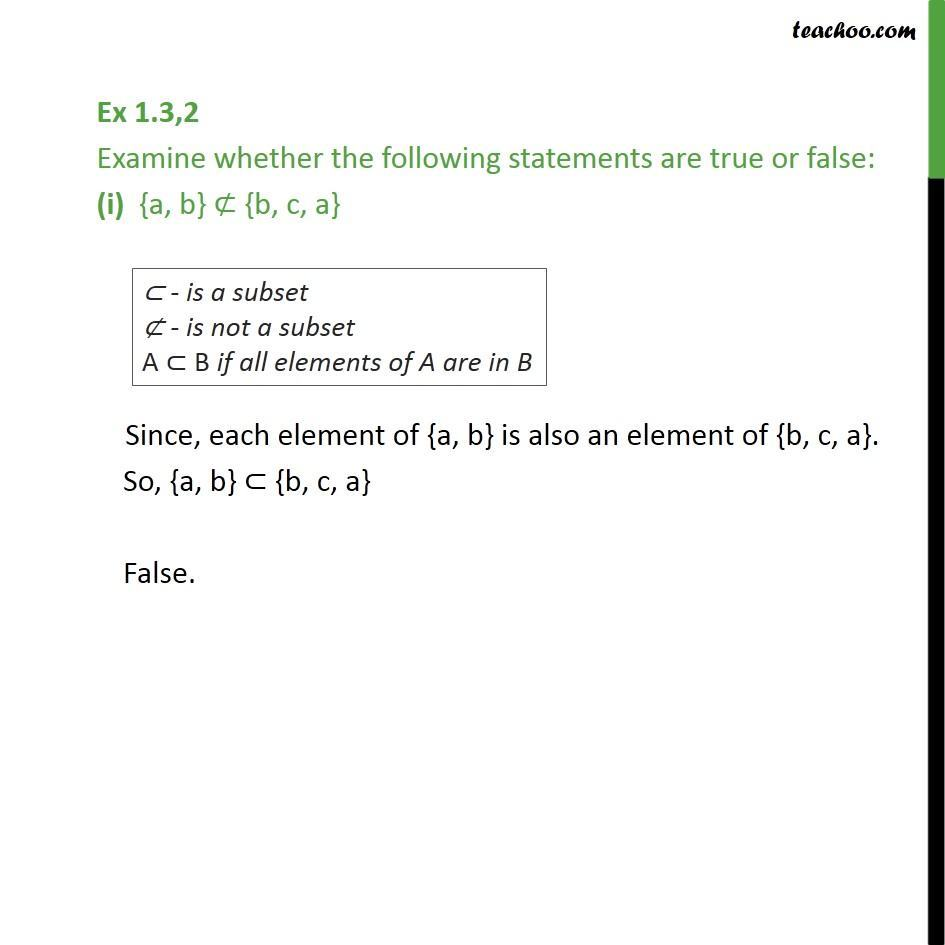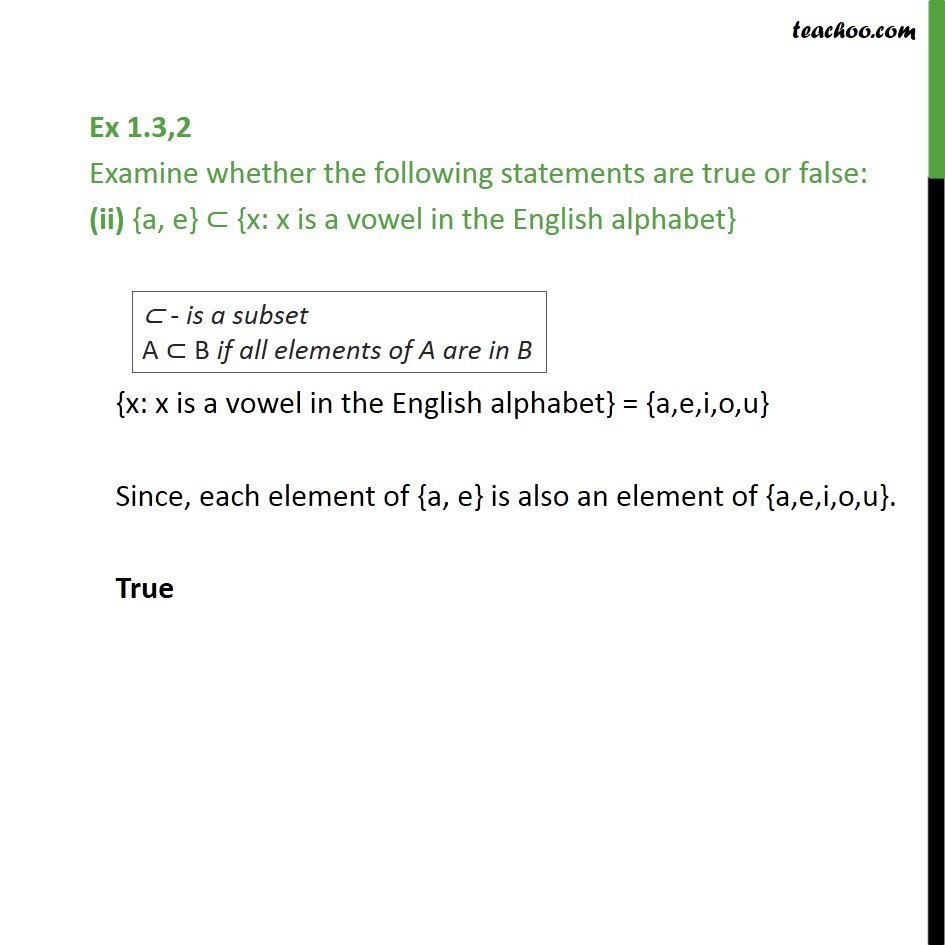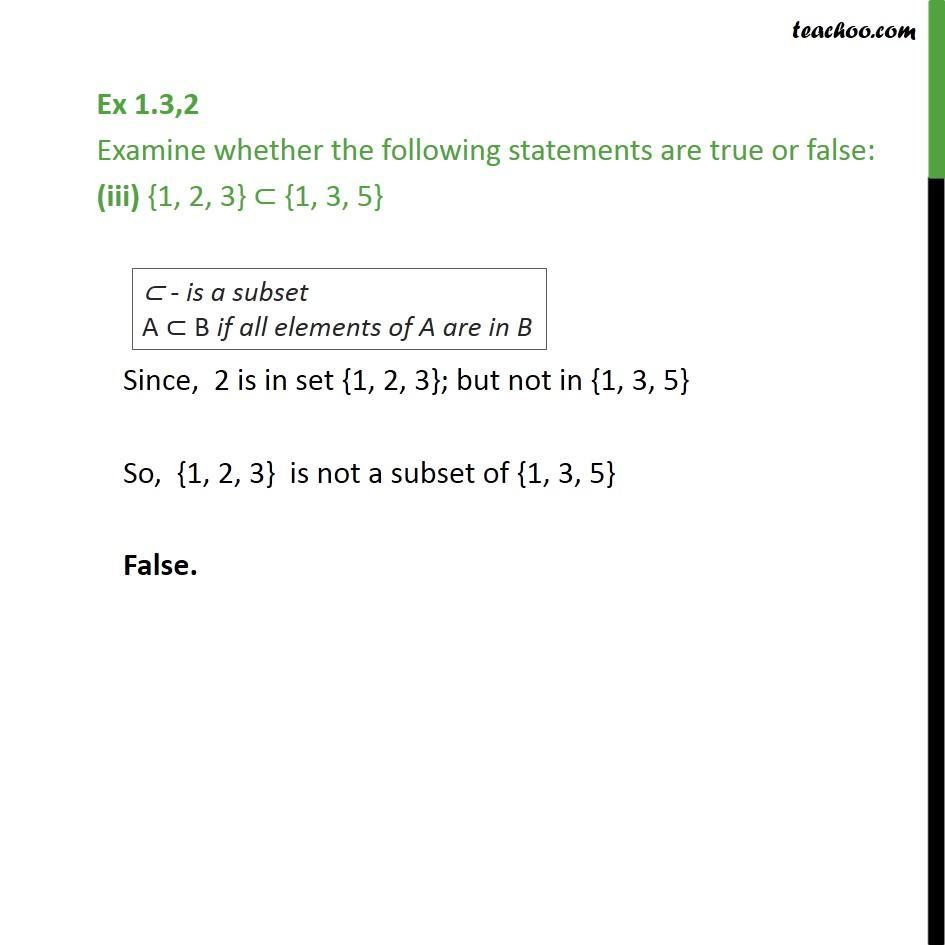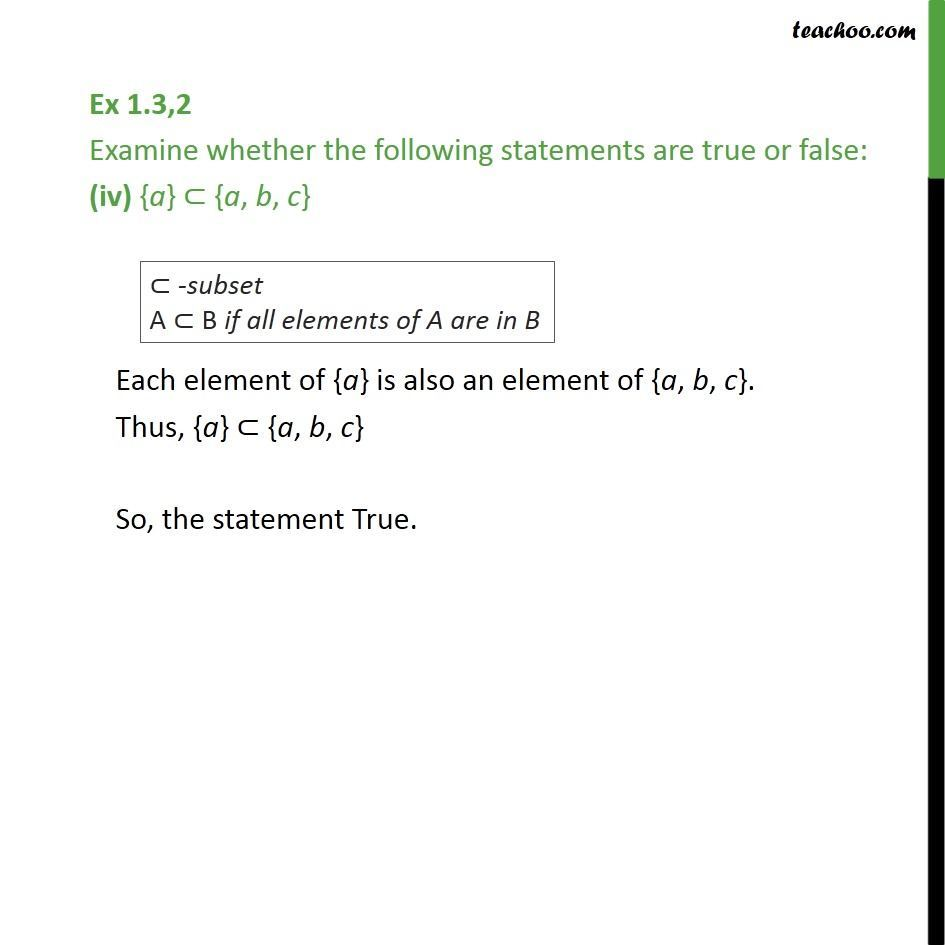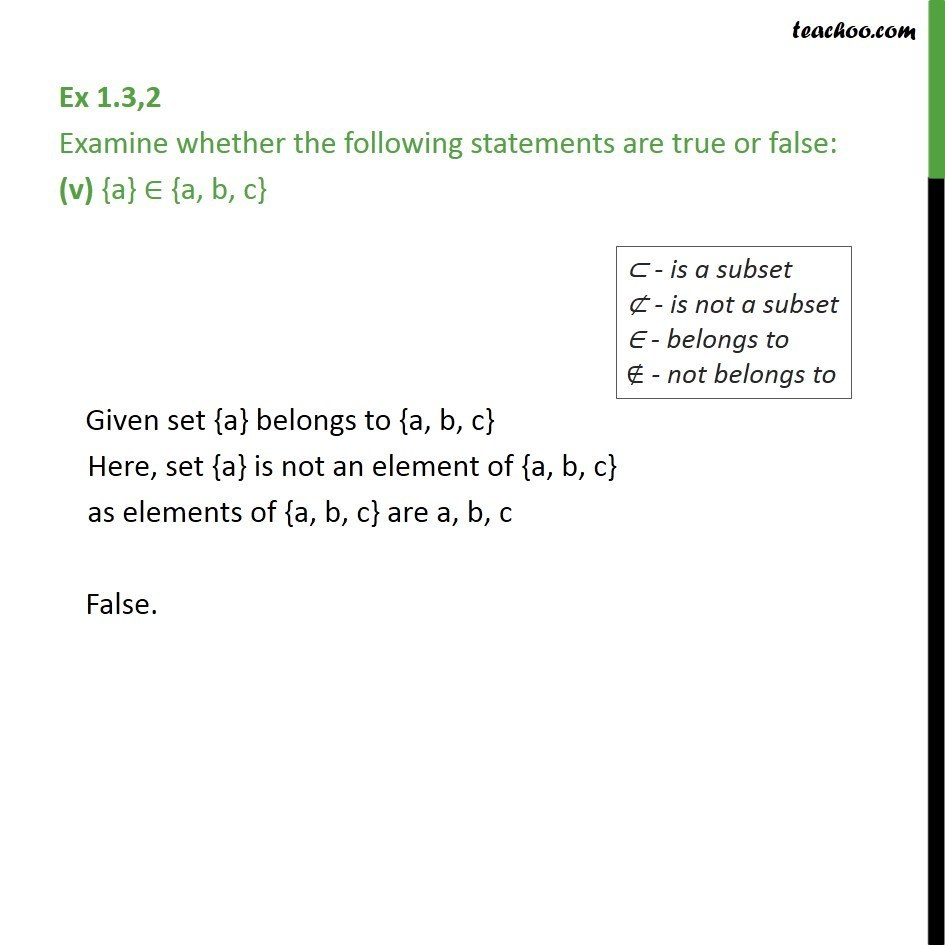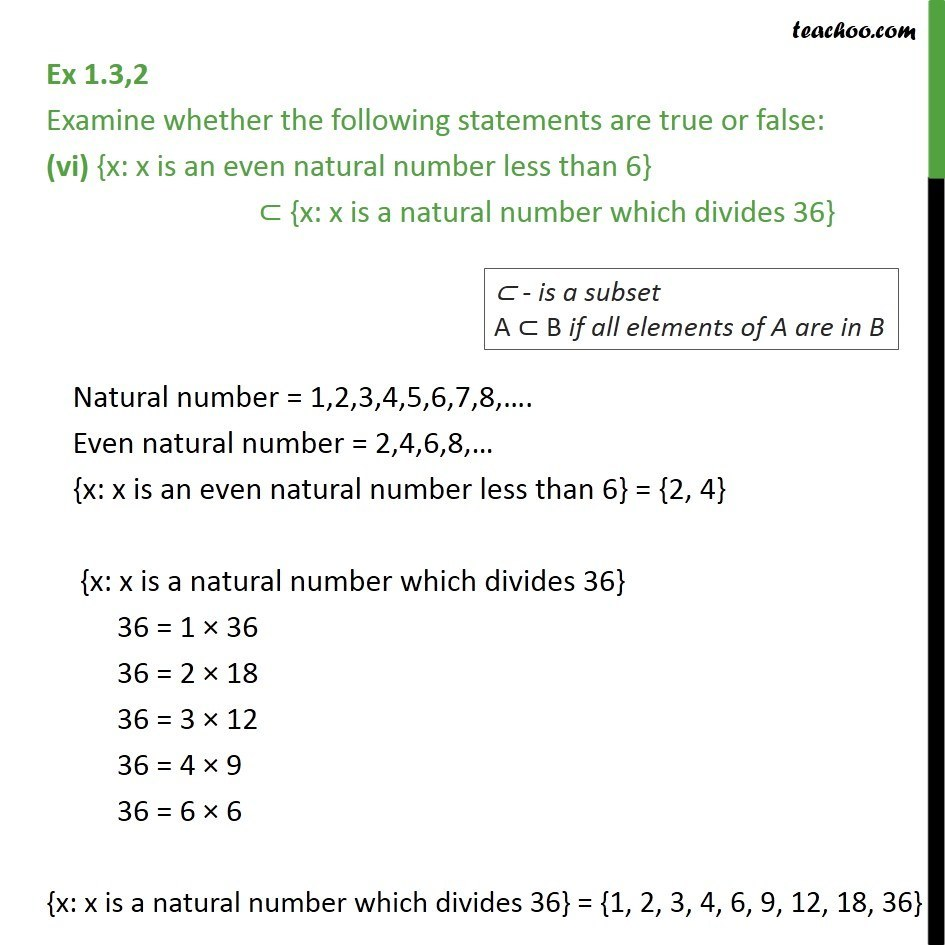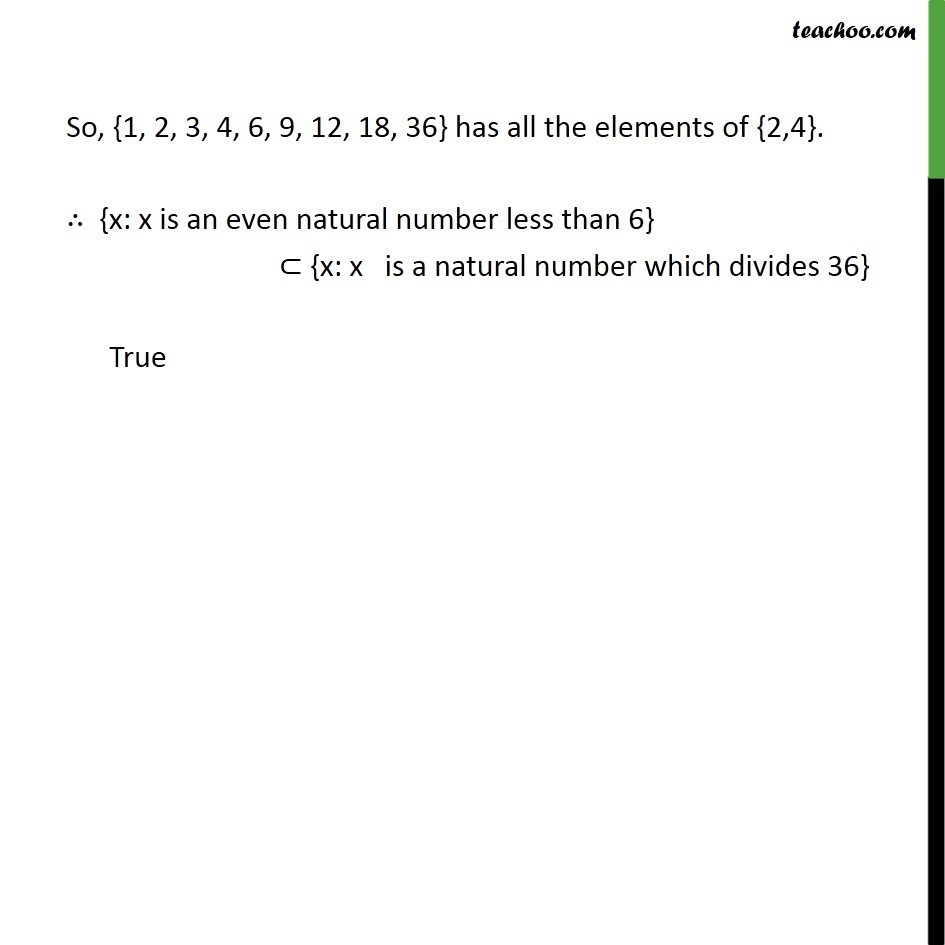1. Chapter 1 Class 11 Sets
2. Concept wise
3. Subset

Transcript

Ex 1.3,2 Examine whether the following statements are true or false: (i) {a, b} ⊄ {b, c, a} Since, each element of {a, b} is also an element of {b, c, a}. So, {a, b} ⊂ {b, c, a} False. Ex 1.3,2 Examine whether the following statements are true or false: (ii) {a, e} ⊂ {x: x is a vowel in the English alphabet} {x: x is a vowel in the English alphabet} = {a,e,i,o,u} Since, each element of {a, e} is also an element of {a,e,i,o,u}. True Ex 1.3,2 Examine whether the following statements are true or false: (iii) {1, 2, 3} ⊂ {1, 3, 5} Since, 2 is in set {1, 2, 3}; but not in {1, 3, 5} So, {1, 2, 3} is not a subset of {1, 3, 5} False. Ex 1.3,2 Examine whether the following statements are true or false: (iv) {a} ⊂ {a, b, c} Each element of {a} is also an element of {a, b, c}. Thus, {a} ⊂ {a, b, c} So, the statement True. Ex 1.3,2 Examine whether the following statements are true or false: (v) {a} ∈ {a, b, c} Given set {a} belongs to {a, b, c} Here, set {a} is not an element of {a, b, c} as elements of {a, b, c} are a, b, c False. Ex 1.3,2 Examine whether the following statements are true or false: (vi) {x: x is an even natural number less than 6} ⊂ {x: x is a natural number which divides 36} Natural number = 1,2,3,4,5,6,7,8,…. Even natural number = 2,4,6,8,… {x: x is an even natural number less than 6} = {2, 4} {x: x is a natural number which divides 36} 36 = 1 × 36 36 = 2 × 18 36 = 3 × 12 36 = 4 × 9 36 = 6 × 6 {x: x is a natural number which divides 36} = {1, 2, 3, 4, 6, 9, 12, 18, 36} So, {1, 2, 3, 4, 6, 9, 12, 18, 36} has all the elements of {2,4}. ∴ {x: x is an even natural number less than 6} ⊂ {x: x is a natural number which divides 36} True

Subset